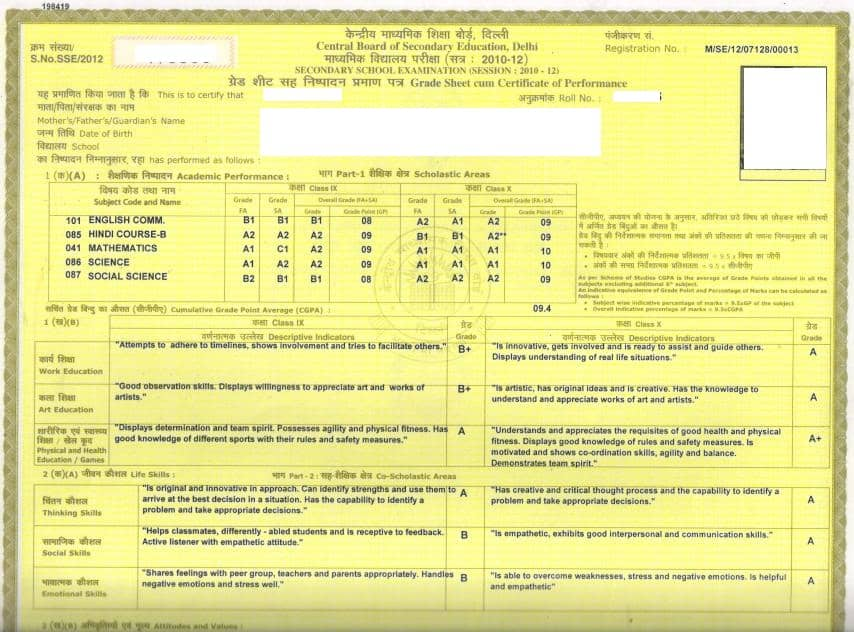# CGPA Calculator How to get Marks from grades## myCBSEguide App

CBSE, NCERT, JEE Main, NEET-UG, NDA, Exam Papers, Question Bank, NCERT Solutions, Exemplars, Revision Notes, Free Videos, MCQ Tests & more.

## What is CGPA?

It stands for Cumulative Grade Point Average. Here is CGPA calculator.

Definition: It is the average of grading points obtained in all subjects excluding the 6th subject as per CBSE scheme.

## CGPA Calculator How to get Marks from grades

In class 10, CBSE there is a grading system. To get an idea about your subject wise and overall percentage of marks and to calculate marks/ percentage from your grades and CGPA, the given table is helpful. Let’s understand it.

### CBSE is using 9 point grading system as below :

 Sr. MARK RANGE GRADE GRADE POINT 1 91-100 A1 10 2 81-90 A2 9 3 71-80 B1 8 4 61-70 B2 7 5 51-60 C1 6 6 41-50 C2 5 7 31-40 D 4 8 21-30 E1 – 9 0-20 E2 –

Individual grades and grade points will be given for each subject. Students will also get overall grade point for five subjects. This overall grade point is called CGPA (cumulative grade point average) and it can be used to calculate estimated overall percentage of marks obtained by the student.

The above formula given by CBSE to convert grades into marks and percentage is only an approximation and not exact.

## CBSE CCE CGPA & Overall Percentage Calculator

 CGPA & Overall Indicative Percentage CGPA INDICATIVE PERCENTAGE

## Here we added a sample result of class 10 for better understanding### Calculation of Percentage for one subject : Grade Point X 9.5

Example : suppose a student get B1 in Hindi and his grade point is 8. Now his estimated percentage in Hindi will be 8 X 9.5 = 76 percent.

### Calculation of overall percentage : CGPA X 9.5

Example : suppose  CGPA (printed on grade sheet) is 7.8. Now his/her estimated overall percentage will be 7.8 X 9.5 = 74.1 percent.

For more insight on grading system and CGPA do check these articles too :

## Note : Abbreviations used against Result :

QUAL – Eligible for Qualifying Certificate,
EIOP – Eligible for Improvement of Performance,
NIOP – Not Eligible for Improvement of Performance,
N.E. – Not Eligible,
R.W. – Result Withheld,
R.L. – Result Later,
UFM – Unfair means,
SJD – Subjudice?### 14 thoughts on “CGPA Calculator How to get Marks from grades”

1. Will our 9th grades count for our 10th next year?

2. Sir,
I’m passed out 10th in 2016,But now i need provisional mark sheet how i get plase suggest me as soon as possible. Or any other way to get in online i meed only original Mark’s.

Thanking you

3. Thanxxx

5. CGPA se Marks me convert kaise kare?

6. myCBSEguide.com is excellent app

7. WHAT TO DO IF CLASS 10 MARKSHEET IS LOST.
AS THIS THING HAPPENED TO ME . I HAVE PASSED CLASS 10 IN 2015 . PLEASE HELP ME OUT AS THIS IS VERY CRUCIAL TO ME

8. This is a perfect app for studying.

9. I passed inter bipc wit 7.83 what are the total marks for this points

10. I passed inter bipc wit 7.83 what are the total marks for this points

11. I would like to know from you about how to change CGPA in marks.

12. sir i wan to calculate the cgpa for class 10th but my grades are give n in A1,B1 etc and not in grade points how can i calculate it according to the year 2020. And what is the grading point scale in year 2020. pls help me out sir

13. How to convert ICSE percentage into CGPA?

14. 100 percent =how many cgpa